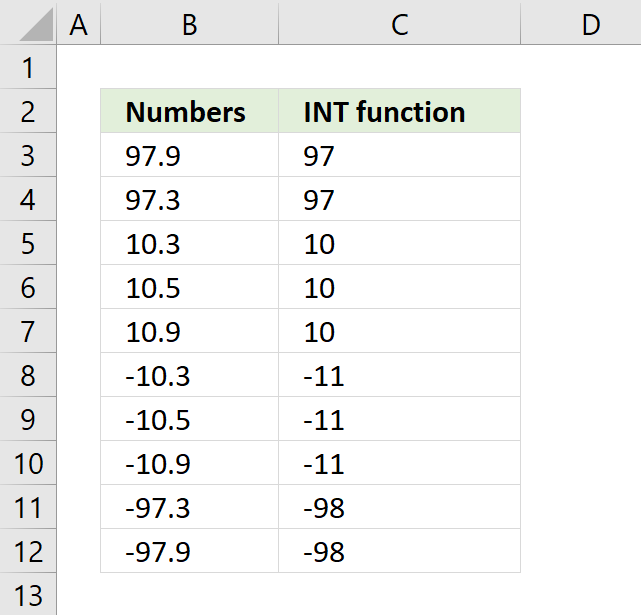Author: Oscar Cronquist Article last updated on August 29, 2018The INT function removes the decimals if the numeric value is above 0 (zero) and returns a negative integer less than or equal to the given argument.

The image above demonstrates how the INT function works. The fractional part of a positive number is removed leaving only the positive integer, example: 10.9 => 10, however, a negative number, example -10.3 is converted to the negative integer that is less than or equal to the argument. -10.3 => -11.

Int ( number )

### Arguments

 number Required. Is a Double or any valid numeric expression. If number is 0 (zero) then 0 (zero) is returned.

```Sub Macro1()